# Traveling Wave Divider Secrets Revealed

Click here to go to our original page on traveling wave dividers

New for September 2019. The traveling wave divider (TWD) was first published in 1980:

Bert and Kaminsky, The Traveling Wave Power Divider/Combiner, IEEE MTT-S, May 1980.

There were several networks described in that paper, we are specifically discussing "Type B" on this page. Like the 1980 paper, we are interested in equal split dividers, but of course you can make different power ratios.  Rather than working out the math for the more general case, we suggest you just use an optimization function.

The outputs of a TWD are in quadrature, as you move down the line each output is 90 degrees longer and has 90 degrees more phase at center frequency. The phase errors from quadrature over frequency can be sizable.  But if you use a traveling wave divider on the input of an SSPA, and the same network as a combiner on the output, the phase errors cancel out. There is one way to screw this up: you need to turn the output combiner to ensure that the signal path doesn't double back on itself. Maybe we need to draw a picture of that.  Do THIS:

Input ---->--------------------------------------------------------------

Amp            Amp            Amp              Amp

------------------------------------------------------------------------------> Output

Not THIS:

Input ---->-------------------------------------------------------------

Amp            Amp            Amp              Amp

Output < --------------------------------------------------------------

## Four-way traveling wave divider

The smallest TWD you can make is a two-way, and it is trivial: just a two-way Wilkinson with an extra 90 degree transmission line on one leg.

Let's start with a four-way TWD. The line impedances and isolation resistor calculations are done from the farthest leg to the nearest leg, and most of the parameters are predetermined as soon as you pick Z0.  It turns out, there are only three degrees of freedom in the ideal case:

1. You can play with the input transformer to match the divider over a very wide band. Theoretically you can match it over infinite bandwidth, with an infinitely long transformer; good luck with that.  One bad-ass way to match it is the Klopfenstein taper, your friends will all be jealous when you present that idea at the design review.  Unfortunately the ultimate limitation is that you can't change the output match and isolation bandwidths. The longer the input transformer, the higher the loss.
2. You can change the lengths of the arms in multiples of quarter-wavelengths. Of course, this will increase the loss.
3. The impedance of the first legs can be changed, so long as the ratio 1:(N-1) is maintained.  In a four-way, Z1B must be 3xZ1A.

Here's a schematic we generated in Microwave Office.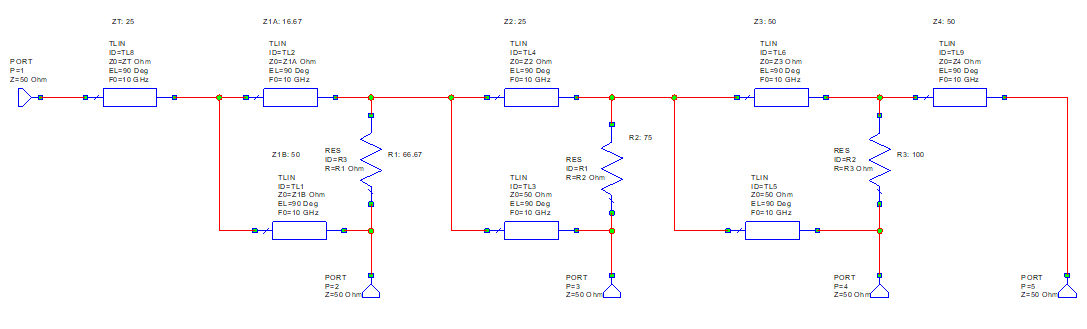Four-way traveling wave divider

Below are equations for the arm impedances and isolation resistor values, which we embedded in the Microwave Office schematic. The "prime" notation indicate the impedance that you would see looking to the right of different nodes. You need to calculate Z1Aprime and Z1Bprime to arrive at the input impedance, Z1prime.  Then the transformer impedance ZT is calculated to match the network.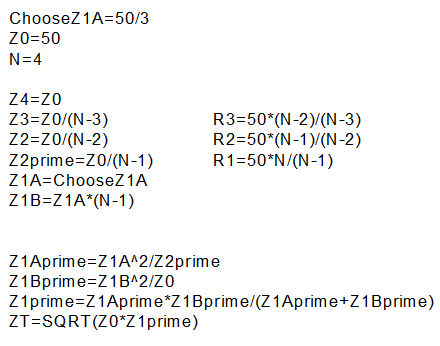The isolation resistors formulas are apparent when you look at the odd mode, and it is well described in the paper so we won't get to it here.  At the final split you see the normal 100-ohm Wilkinson resistor, then they gradually get smaller.  The reason is also explained on our unequal split Wilkinson page.

Note that in the above set of equations a pattern is apparent : Z3=Z0/2, Z2=Z0/3... if we continue the pattern, Z1 becomes Z0/3 (16.7 ohms in fifty ohm system) and you get the following results. Yes, it kind of stinks, the bandwidth is parabolic, and the input match is narrow.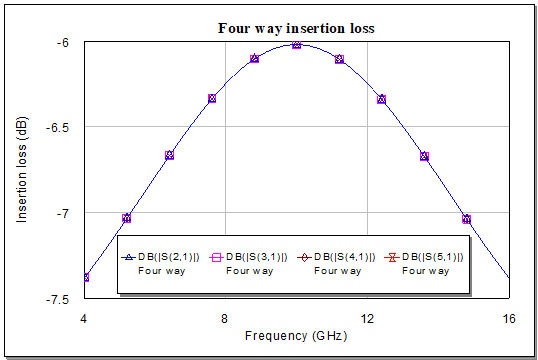Four-way insertion loss with ZA=16.7 ohms

Note that the model used lossless transmission lines, so the losses are all predicted to be identical. In reality you will see that loss increases as you move down the divider.  You can always equalize the losses with a little bit of optimization, allowing the impedances and resistors to drift away from ideal values.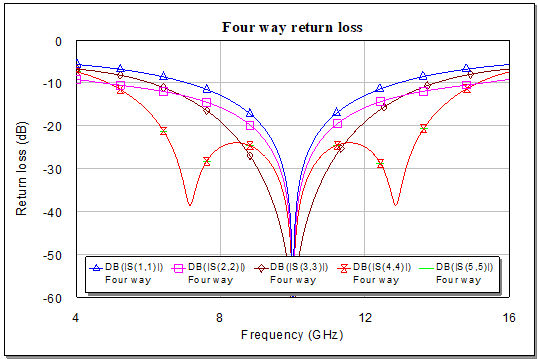Four-way return loss with ZA=16.7 ohms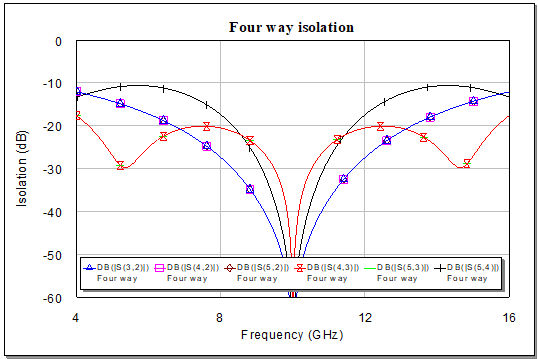Four-way isolation with ZA=16.7 ohms

## Optimized four-way, traveling wave divider

We added an optimization function to improve the insertion loss flatness and let loose the infinite monkeys. Z1A increased from 16.7 to 23.5 ohms.  In the original paper the authors came up with 21.5 ohms, beauty is in the eye of the beholder. Here's the optimized schematic, note that the only changes are Z1A, Z1B and ZT.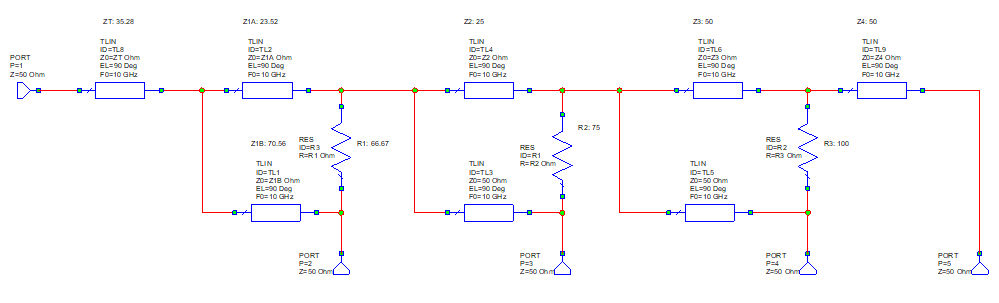Optimized four-way traveling wave divider

If you can't read the schematic this will make it clear: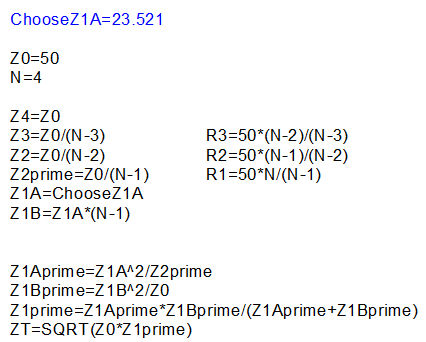It sure did improve...  Behold, 40% bandwidth!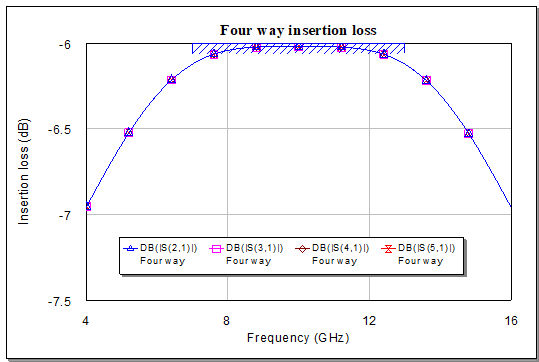Four-way insertion loss with Z1A=23.5 ohms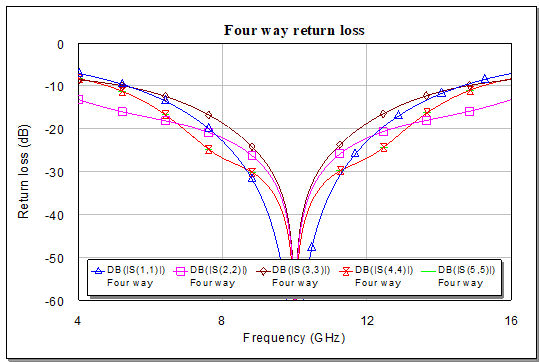Four-way return loss with Z1A=23.5 ohms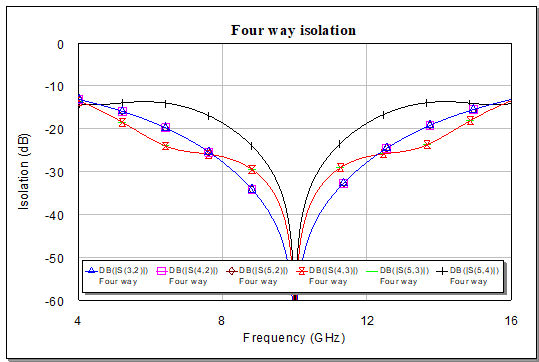Four-way isolation with Z1A=23.5 ohms

Speaking of "beholding", here is Dr. Weird, somewhere on the South Jersey Shore, with many wonders for you to behold:

Dr. Weird

## Five-way traveling wave divider

Now let's look at a five-way version.  Following the Z-pattern, the impedance of the first leg Z1A is 12.5 ohms (Z0/4).  Whoops, this is getting really low! This is the major limitation of the traveling wave divider, the more outputs, the lower the minimum impedance required.  In practice, this is why you will never see a TWD higher than N=4.  But let's continue our look at N=5 for fun.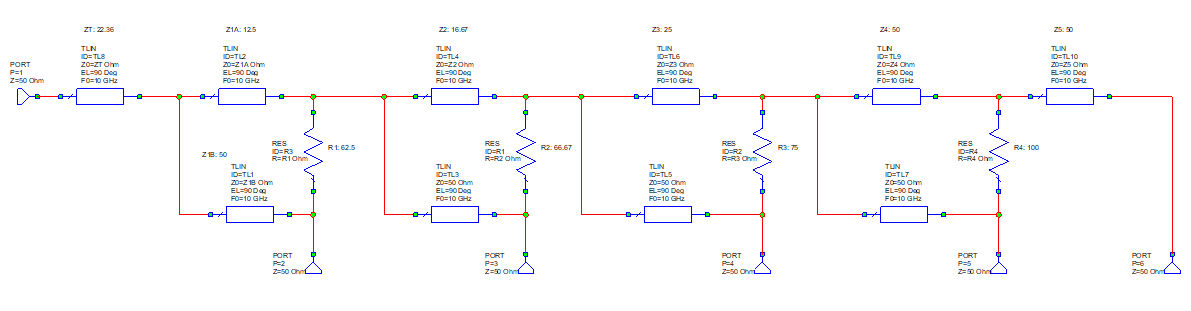Five-way traveling wave divider

Here is a graphic of the equations used in the schematic.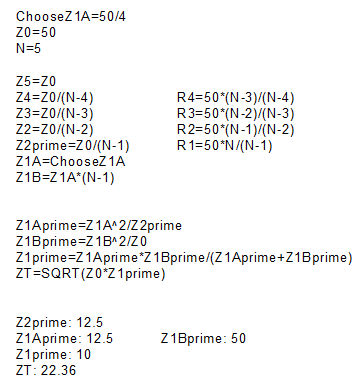Again the bandwidth is peaked up, and exactly no one is gonna want this response.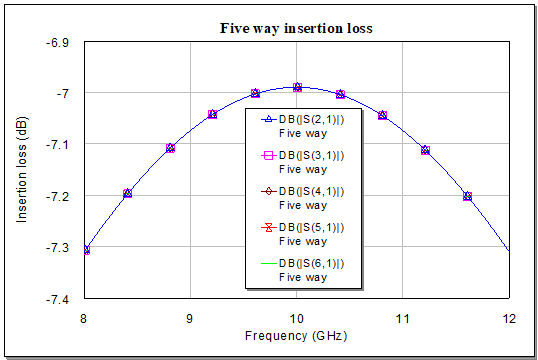Five-way insertion loss with Z1A=12.5 ohms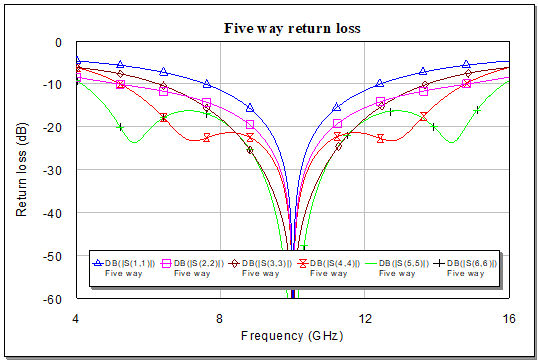Five-way return loss with Z1A=12.5 ohms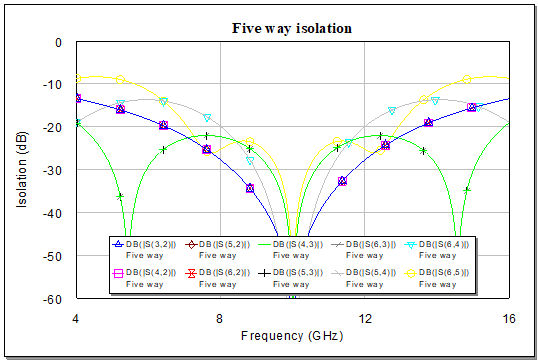Five-way isolation with Z1A=12.5 ohms

## Optimized five-way traveling wave combiner

Now let's optimize Z1A and see what we get.  It ends up at 18.7 ohms (easier to fabricate than 12.5 ohms!), with Z1B at 4X that value following the rule we described.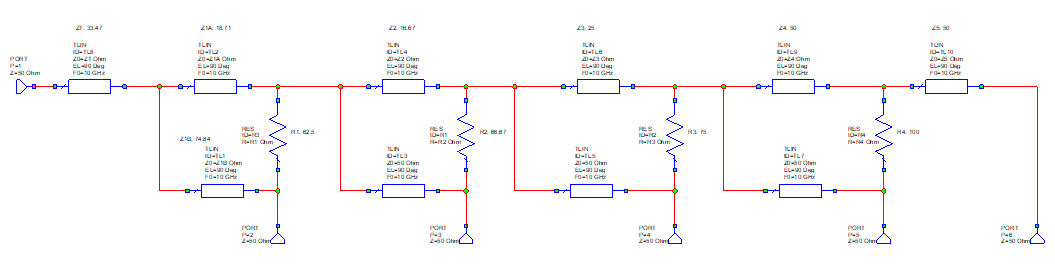Optimized five-way traveling wave divider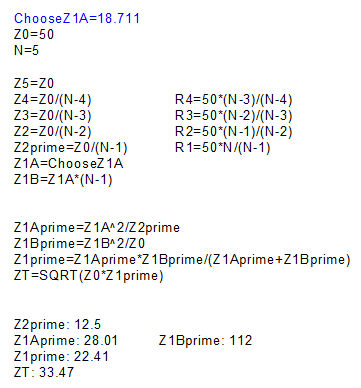Yay, the insertion loss has a flat spot over ~40% bandwidth!Five-way insertion loss, Z1A=18.7 ohms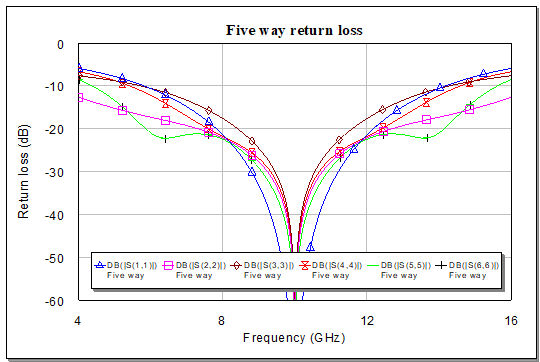Five-way return loss, Z1A=18.7 ohms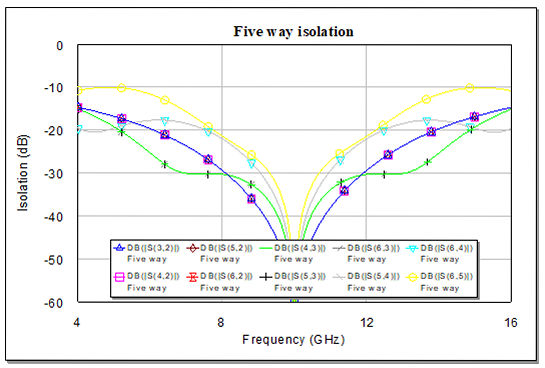Five-way isolation, Z1A=18.7 ohms

## Five-way TWD microstrip layout

We converted the ideal transmission lines to microstrip (10 mil alumina) and generated a layout of the main arm.  First, here is the version with Z1A=12.5 ohms.  The lines segments are ZT, Z1A, Z2, Z3, Z4 and Z5. Notice how wide Z1A is, its width is getting dangerously close to a quarter-wavelength. By the time you add the output arms and resistors and EM it, you will be in misery.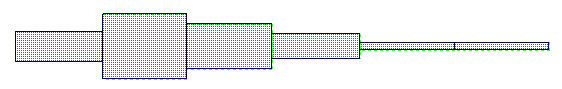Five-way microstrip layout, Z1A=12.5 ohms

Now lets look at the optimized version.  It certainly gives a little more hope that you could produce it.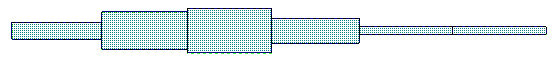Five-way microstrip layout, Z1A=18.7 ohms

Below is a summary of line impedeances for TWDs up to eightway.  The Z1A values are for Z0/(Z-1), not the optimum values. It seemed like a waste of time to create an eightway and optimize it, as there is no practical way to produce it.

## Summary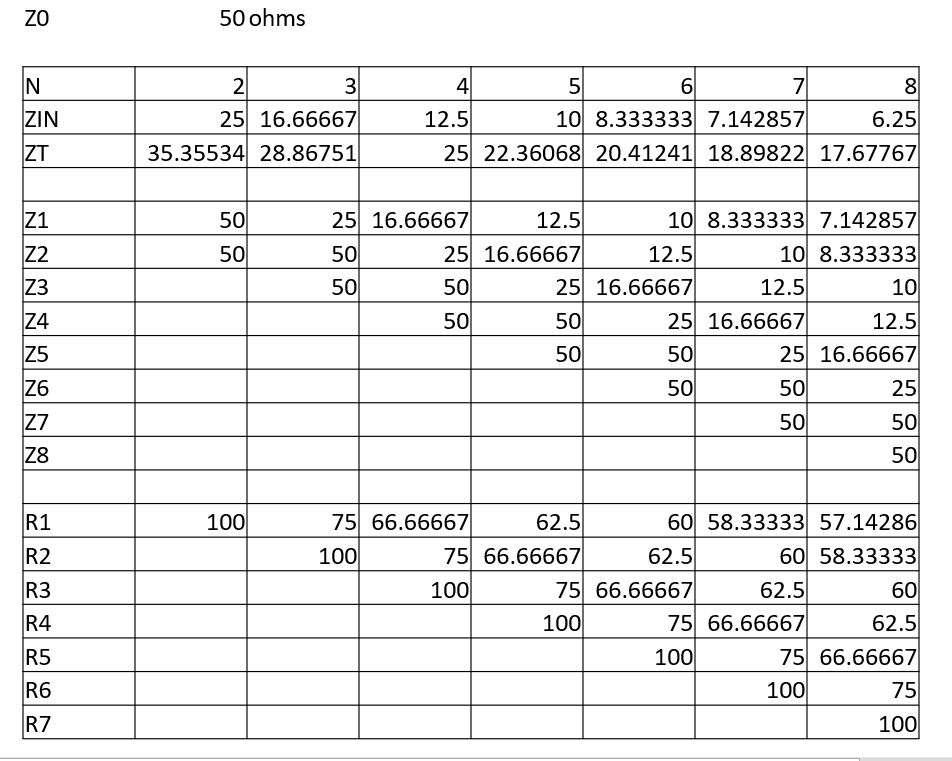Comments or confused? Drop us a line and we'll update the page!

Author : Unknown Editor